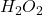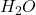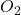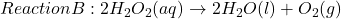## Reaction B: 2 H2O2 (aq) + 2 H20 (L) + O2 (g) 10. Identify the reactants in Reaction B. 11. Identify the products in Reaction B.<

Question

Reaction B: 2 H2O2 (aq) + 2 H20 (L) + O2 (g)
10. Identify the reactants in Reaction B.
11. Identify the products in Reaction B.
12. How many molecules are present on the reactant side of the equation?
13. How many molecules are present on the product side of the equation?

in progress 0
6 months 2021-08-14T05:36:54+00:00 1 Answers 14 views 0

## Answers ( )

1. Answer: 10. The reactant in Reaction B is.

11. The products in Reaction B areand.

12. There are 2 molecules present on the reactant side of the equation.

13. There are 3 molecules present on the product side of the equation.

Explanation:

The given reaction equation is as follows.Reactants are the species present on the left side of a chemical equation.

Products are the species present on the right side of a chemical equation.

Therefore, the reactant in Reaction B is. As 2 is coefficient ofso, 2 molecules are present on the reactant side of the equation.

The the products in Reaction B areand. The coefficient ofis 2 and coefficient ofis 1. Hence, there are total (2 + 1) equals to 3 molecules present on the product side of the equation.

Thus, we can conclude that:

The reactant in Reaction B is.

The products in Reaction B areand.

There are 2 molecules present on the reactant side of the equation.

There are 3 molecules present on the product side of the equation.